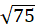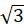# Simplify Square Root Calculator

## Why use an online simplify square root calculator?

There are some square roots that are really difficult on first look and take a lot of time in finding out the right solution.

Therefore, Mycalcu provides simplify square root calculator to solve all such difficult and time-consuming problems for you.

Solving equations and problems with an online calculator is extremely useful because it will simplify them for you.

Feel free to use this online tool without logging in or paying anything.

## How does it work?

Simplifying a difficult square root equation is not that much hard as it is considered to be. In order to simplify the difficult square roots, you have to factorize the number inside the given root.

After factorizing into smaller numbers, pull the perfect squares out of the radical sign and your problem is solved.

For example,In the first step factorize the 75: 5 × 5 × 3

You can see that there comes a perfect square therefore answer will be

5### How to use simplify square root calculator?

Enter the given square root correctly and after factorization of that number, you will get the solution